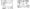# CSVTU Exam Papers – BE I Year – Engineering Graphics– 2006

BE (1st Semester)

Examination – 2006

Engineering Graphics

Q1  (a) On a map, the distance between two points is 14 cm. The real distance between them is 20 km. Draw a diagonal scale of the map to read kilometers and hectameters, and to measure up 25 km. Show a distance of 17.6 km. on this scale.

(b)  Show by means of a drawing that when the diameter of the directing circle is twice that of the generating circle, th hypocycloid is a straight line. Take the diameter of ft generating circle equal to 50 mm

(c) An inelastic string 145 mm long, has its one end attached to the circumference of a circular disc of 40 mm diameter. Draw the curve traced out the other and of the string, when it completely wound around the disc, keeping the string always tight.

Q2 (a) A point C is 15 mm above H.P. and 45 mm in front of V.P. In what quadrant another point D lies if the .distance between vertical projection of  C and D be equal 60 mm and the distar between the projectors through C and D be 40 mm and point D is 40 mm in front of V.P.

(b)The top view of a 75 mm long line AB meansures 65 mm, whith the length of its front view is 50 mm. Its one end A is in the H  and 12 mm in front of the V.P. Draw the projections of AB an determine its inclinations with the H.P. and V.P.

(c) The projector of the ends of a line AB are 5 cm apart. The or A is 2 cm. above the H.P. and 3 cm in front of the V.P. The or B is 1 cm below the H.P. and 4 cm behind the V.P. Determine the true length and traces of AB, and is inclinations with the  two  planes.

Q3  (a) Draw the projections of a regular pentagon of 40 mm side having its surface inclined at 30° to the H.P. and a side paral to the H.P. and inclined at an angle of 60° to the V.P.

(b) A square pyramid, base 38 mm side axis 50 mm long, is free suspended from one of the corners of its base. Draw projections, when the axis as a vertical plane makes an angle of 45° with the V.P.   (c) A tetrahedron of 65 mm long edges is lying on the ground on one of its faces, with an edge perpendicular to the V.P. It is cut by a section plane which is perpendicular to the V.P. So that the true shape of the section is an isosceles triangle of base 50 mm long and altitude 40 mm. Find the inclination of the section plane with the H.P. and draw the front view, section top view and the true shape of the section.

Q4 (a) Draw the projection of a cone resting on the ground on its base and how on them, the shortest path by which path by which a point P, starting from a point on the circumference of the base and moving around the cone will return to the same point, Base of cone 65 mm diameter, axis 75 mm long.

(b)  Draw the isometric view of the figure given below.(c) A right regular hexagonal prism, edge of base 20 mm and height 50 mm, has a circular hole of 20 mm diameter, drilled centrally through its, along its axis, Draw its isometric projection.

Q5 (a) What are the benefit and limitation of CAD?

(b) Write any five basic commands of Auto CAD Also indicate the various options available in each command.

(c) What is the use of layers in making drawing in Auto CAD?